# SambaPOS crashes when used the same action twice in a rule

I have a Delivery Charge action which I use twice in a rule as follows:

Delivery Charge
Constraint: {PLAIN TOTAL} < 12
Amount: 2

Delivery Charge
Constraint: {PLAIN TOTAL} >= 12
Amount: 0

When I add items to the ticket and go over 12, SambaPOS crashes, somehow because of these two constraints. What is wrong?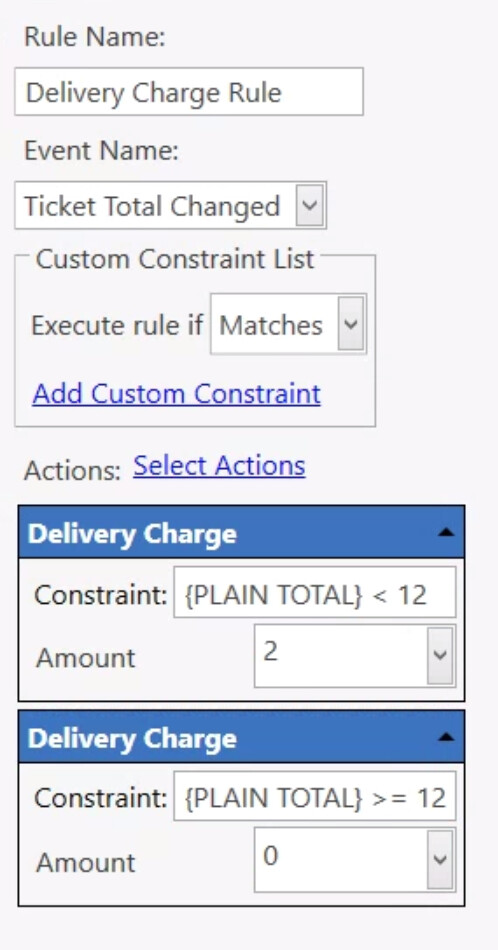When replaced with a show message action it works fine and does not crash.

Edit: I noticed It triggers twice as if both constraints are true.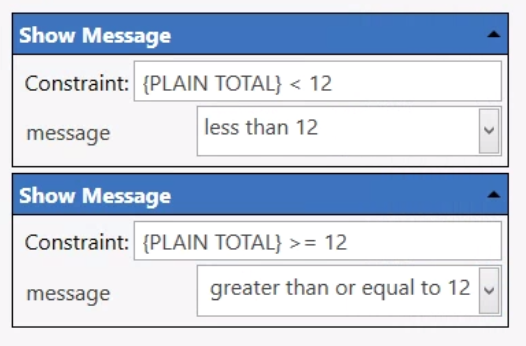Not sure but can you try

``````'[={PLAIN TOTAL}]' < '12'
``````

To be fair I dont really know what PLAIN TOTAL even is. I never used it and it never showed anything on the ticket templates when I tested itTry this, this seems to work

``````'[=TN('{TICKET TOTAL}')]' < 12
or
'[=TN('{PLAIN TOTAL}')]' < 12
``````

No, actually {PLAIN TOTAL} works fine.

I noticed, adding/removing a delivery charge causes an infinite loop. I have an idea why, let me check if I’m right.

Just tested it now, PLAIN TOTAL actually gives a value if the TICKET TOTAL has been changed using Discounts/Round or possibly other calculations.

PLAIN TOTAL is not defined unless it’s different from TICKET TOTAL. Is that right?

Is there a way to force PLAIN TOTAL to return a value? (Looking another thread, I tried putting it in brackets like [{PLAIN TOTAL}].)

I tried to set Amount to non-zero for all conditions and it works because the TICKET TOTAL is always different than PLAIN TOTAL, so PLAIN TOTAL is defined.

Now, maybe I could check if PLAIN TOTAL is defined in the constraint, but is there anything I can do to simply force PLAIN TOTAL is always defined?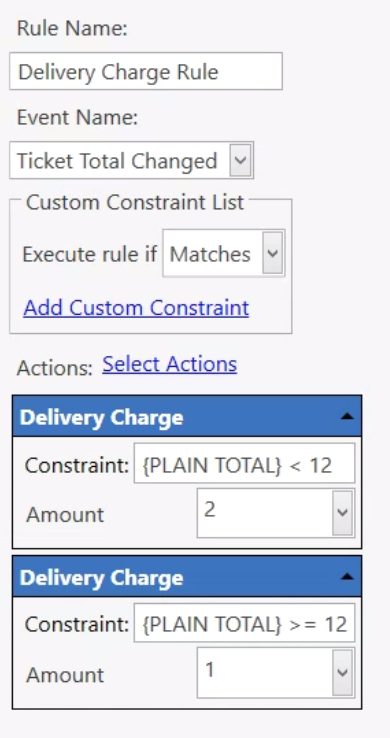Use {TICKET PLAIN SUM:} That forces it.

1 Like

Thank you! I hope it will work but at the moment I get {TICKET PLAIN SUM:} as the message not the sum. Here is how I tested: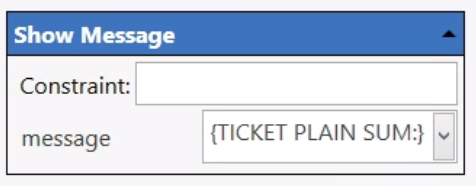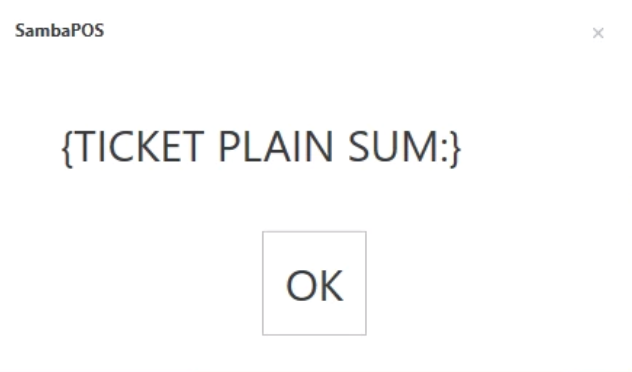`{PLAIN TOTAL} > 12` and `{PLAIN TOTAL} >= 12` can cause a StackOverflow in the ClearScript engine when it’s trying to evaluate the expression under the right circumtances.

Wrapping `{PLAIN TOTAL}` in a to-number expression seems to avoid the StackOverflow:

``````[=TN('{PLAIN TOTAL}')] < 12
[=TN('{PLAIN TOTAL}')] >= 12``````

Can you confirm {TICKET PLAIN SUM:} doesn’t exist at all?

This also does not work. PLAIN TOTAL is not defined if it’s the same as TICKET TOTAL.

Here is my current solution. (Might not be a good one. I’m not sure TICKET TOTAL - DISCOUNT TOTAL will always be equal to PLAIN TOTAL. Guess not.)

Delivery Charge
Constraint: ({TICKET TOTAL} - {DISCOUNT TOTAL}) < 12
Amount: 2

Delivery Charge
Constraint: ({TICKET TOTAL} - {DISCOUNT TOTAL}) >= 12
Amount: 0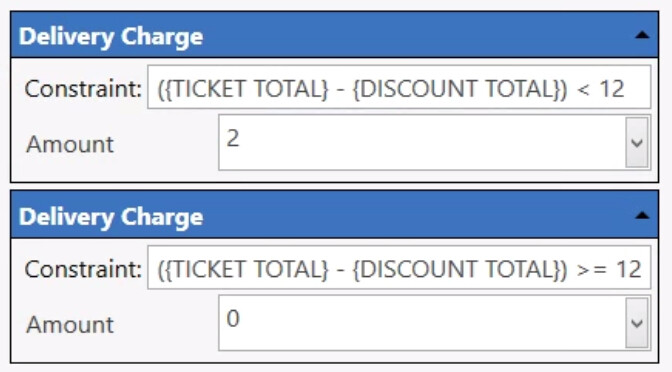Change your calculation format to:
`[={TICKET TOTAL} - {DISCOUNT TOTAL}]`

The way you have it structured would look something like this:
(10.00 - 3.00) > 12
Both sides of the operator would never be equal.

This should return the ticket total sales minus any discounts:

`[=(TN('{PLAIN TOTAL}') - (TN('{DISCOUNT TOTAL}') * -1))]`

Constraints should be like this:

`[=(TN('{PLAIN TOTAL}') - (TN('{DISCOUNT TOTAL}') * -1))] < 12`

`[=(TN('{PLAIN TOTAL}') - (TN('{DISCOUNT TOTAL}') * -1))] >= 12`

1 Like

Was going to say, probably want to wrap them with a TN if doing math or <>

What does <> do?

20chars

Okay, so I missed the part about {PLAIN TOTAL} being undefined under certain circumstances.

So, give this a try:

`[=('{PLAIN TOTAL}' == '' ? '{TICKET TOTAL}' : TN('{PLAIN TOTAL}') - (TN('{DISCOUNT TOTAL}') * -1))] < 12`

`[=('{PLAIN TOTAL}' == '' ? '{TICKET TOTAL}' : TN('{PLAIN TOTAL}') - (TN('{DISCOUNT TOTAL}') * -1))] >= 12`

Was only referring to larger or less than functions.Wasn’t really need to specify < and >, but just have the impression from time using samba that they were more fussy on needing TN where simple math +/- maybe had some intelligence if they did get a number not strictly an interger. No evidence or testing to back that up mind, just feels like have had to go back and add TN when using them moreso than +/-. Probably just coinsidence have usually used them in more complex constraints with other mistakes LOL

Ahh, I thought <> was some super secret squirrel string enclosure for something. Way to get my hopes up.But hey, until recently I thought [=F()] was for Function not Format.